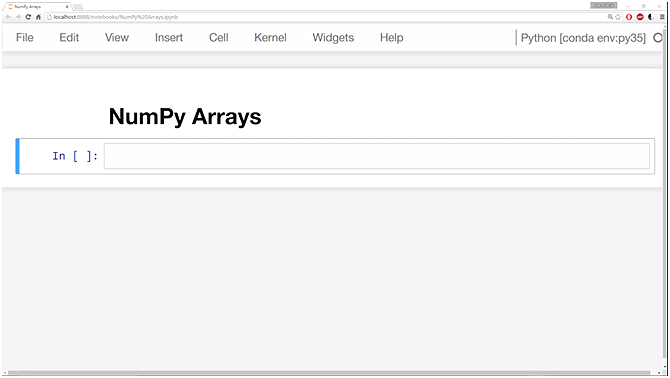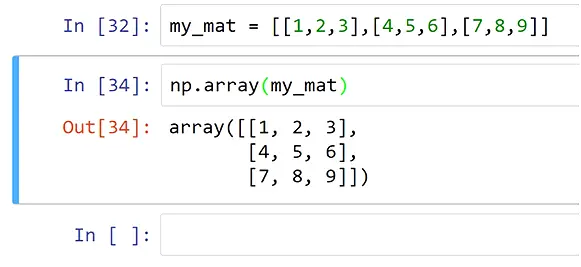## Python Data Science Tutorial Index

Numpy can be abbreviated as Numeric Python, is a Data analysis library for Python that consists of multi-dimensional array-objects as well as a collection of routines to process these arrays. In this tutorial, you will be learning about the various uses of this library concerning data science.

NumPy is a linear algebra library for Python, and it is so famous and commonly used because most of the libraries in PyData's environment rely on Numpy as one of their main building blocks. Moreover, it is fast and reliable.

It is recommended that your system should have Python installed via Anaconda distribution (www.continuum.io or www.anaconda.com ) for making sure all underlying dependencies get sync up with use of conda install. The Python's standard distribution doesn't come with NumPy library module. So for installing Numpy, you have to go to command prompt (Windows users) or terminal (Mac users) and type the following:

```conda install numpy

pip install numpy```

In this chapter, you will be using numpy arrays. Numpy comes in two different flavors. These are:

• Vectors and
• Matrices

Here vectors are 1D (a one-dimensional array of elements), and matrices are 2D (two dimensional) array of elements. It is to be noted that matrices can also hold only either one row or one column as well.

### Numpy Operations and Features

Numpy allows developers to do the following operations:

• Shape manipulation and Fourier transformation
• Logical as well as mathematical operations
• Linear algebra operations using inbuilt functions

### Implement the Numpy Library

So for implementing different Python codes implementing numpy for data science you have to open Jupyter notebook.As you open Jupyter notebook for writing code, you will see this above interface and in the In[ ]: section you have to insert your script. The NumPy arrays will be the keyway of implementing the entire NumPy library. So let us start using NumPy.

``````my_dataList = [2, 4, 6]

import numpy as np

ar = np.array (my_dataList)

ar``````

Output:

``Out[ ]: array ( [2, 4, 6])``

It will return a one-dimensional array.

#### Another Code snippet

``````# assigning nested list, which is a 2D format
my_mat = [[1,2,3], [4,5,6], [7,8,9]]

# Cast the list variable to an array() and use the library np
np.array(my_mat)``````You will notice the output will look something like this (in red):

Here is the output you can see a 2D array having three rows and three columns of values that were assigned earlier to the my_mat variable.